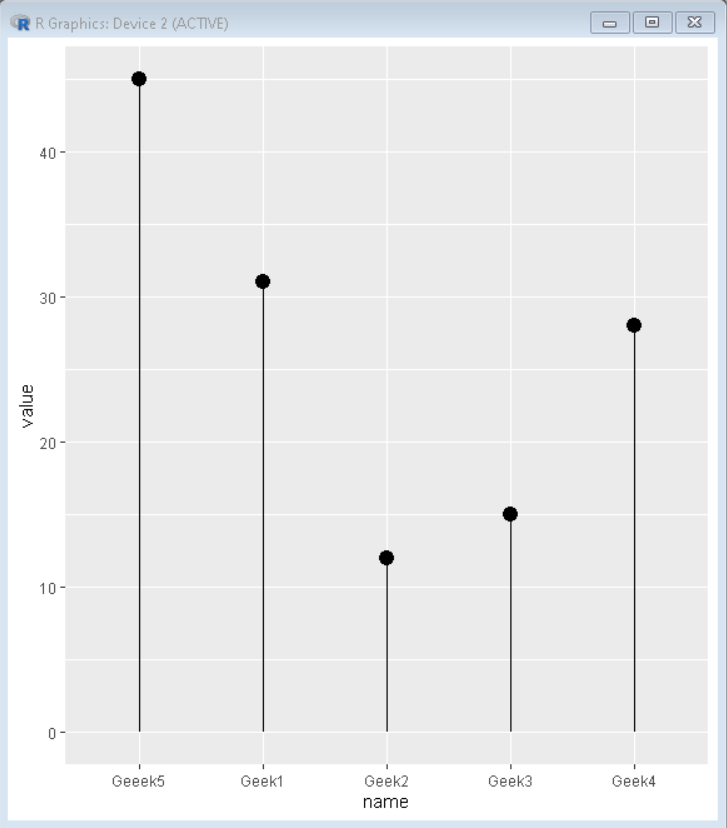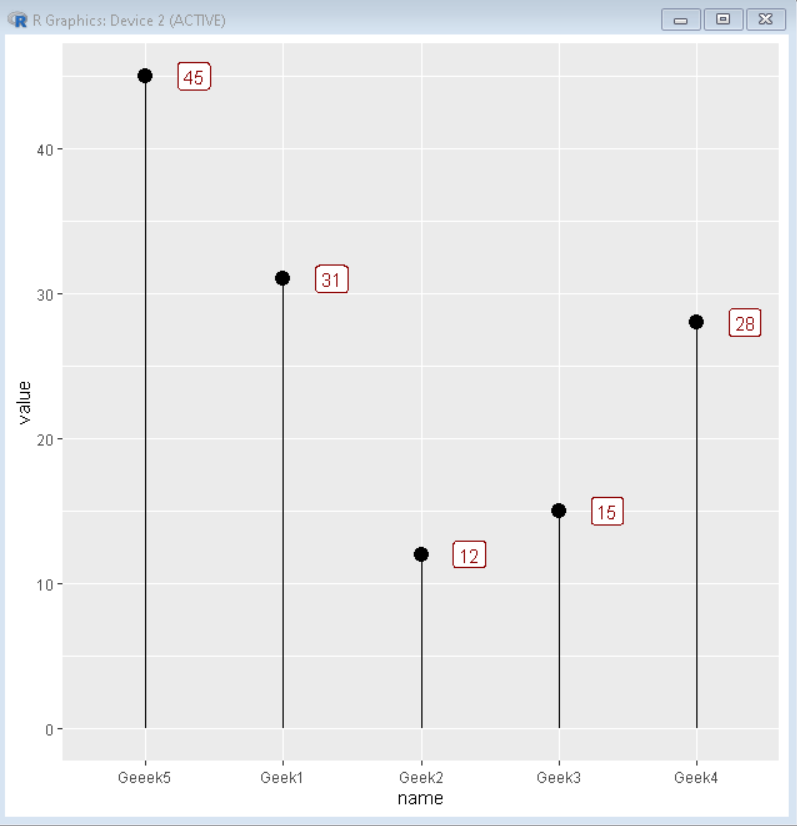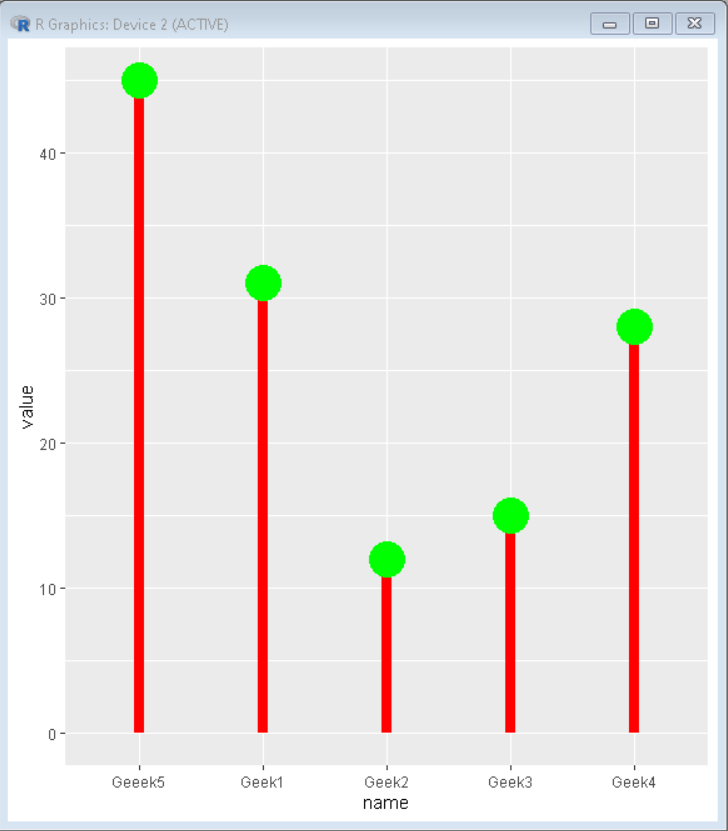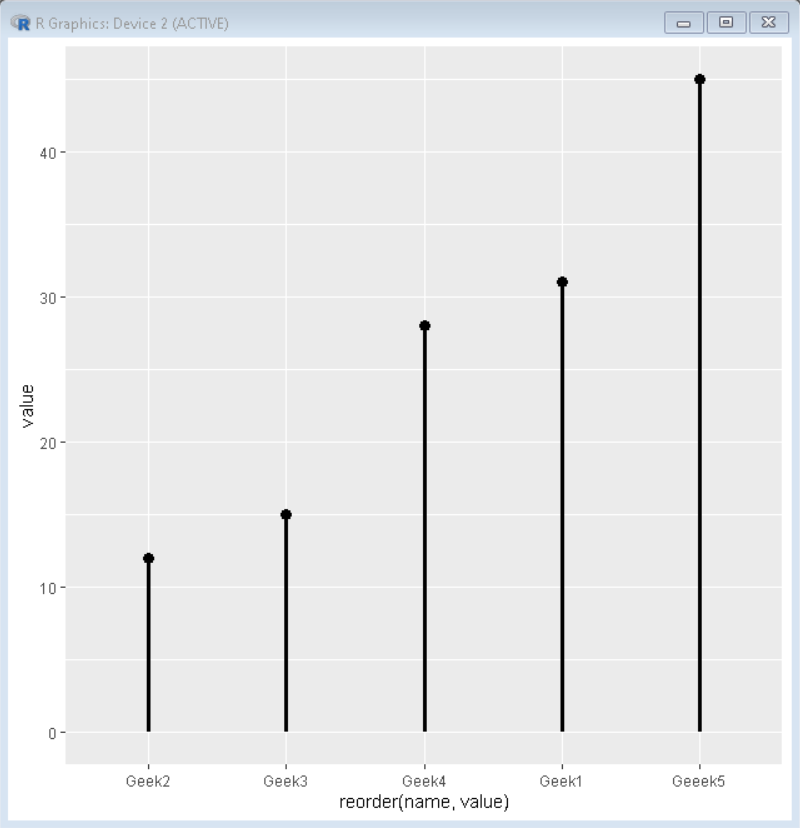How To Make Lollipop Plot in R with ggplot2?

• Difficulty Level : Expert
• Last Updated : 05 Oct, 2021

A lollipop plot is the combination of a line and a dot. It shows the relationship between a numeric and a categorical variable just like a barplot. A lollipop plot can be used at places where a barplot and scatter plot both are required. This single plot helps us visualize the problem better and takes less ink space than traditional barplots.

In ggplot2, we make a lollipop plot by concatenating geom_segment() and geom_point() function.

Syntax: ggplot(data, aes(x=x, y=y)) + geom_segment() +geom_point( )

R

 # Create sample dataset.seed(5642)                             sample_data <- data.frame(name=c("Geek1","Geek2",                                 "Geek3","Geek4",                                 "Geeek5") ,                                                      value=c(31,12,15,28,45))   # Load ggplot2 library("ggplot2") # Create lollipop plotggplot(sample_data, aes(x=name, y=value)) +geom_segment( aes(x=name, xend=name, y=0, yend=value)) +geom_point(size=4)

Output:To add Annotations to ggplot2 lollipop plot, we use geom_label() function:

Syntax: geom_label(aes(name, value, label = signif(value)), colour, nudge_x, size)

Here,

• color: determines the color of annotations
• nudge_x: determines the x shift of annotations
• size: determines the size of annotations

Code:

R

 # Create sample dataset.seed(5642)                             sample_data <- data.frame(name=c("Geek1","Geek2","Geek3","Geek4","Geeek5") ,                          value=c(31,12,15,28,45))   # Load ggplot2 library("ggplot2")   # Create lollipop plot with annotationsggplot(sample_data, aes(x=name, y=value)) +geom_segment( aes(x=name, xend=name, y=0, yend=value)) +geom_point(size=4) +geom_label(aes(name, value , label = signif(value)),            colour = "darkred", nudge_x = 0.35, size = 4)

Output:Customize the Plot

To customize this plot we can change the color of the segment and point using the fill property. We can also change the size of the point using the size property. To add color to geom_segment() we add a color property with the desired color and add size property for the thickness of the segment. To add color to geom_point() we add a color property with the desired color and add size property for increasing the size of the point.

R

 # Create sample dataset.seed(5642)                             sample_data <- data.frame(name=c("Geek1","Geek2","Geek3",                                 "Geek4","Geeek5") ,                          value=c(31,12,15,28,45))   # Load ggplot2 library("ggplot2")   # Create lollipop plot with custom colorsggplot(sample_data, aes(x=name, y=value)) +geom_segment( aes(x=name, xend=name, y=0, yend=value),              color="red", size=3) +geom_point( color="green", size=10)

Output:Reorder lollipop plot

We can reorder the lollipop plot using reorder() function with value as a base.

Syntax: ggplot(sample_data, aes(x=reorder(name,value),y=value))

We add reorder function in the aesthetics parameter to reorder our data frame in ascending order with a base of value.

R

 # Create sample dataset.seed(5642)                             sample_data <- data.frame(name=c("Geek1","Geek2","Geek3",                                 "Geek4","Geeek5") ,                          value=c(31,12,15,28,45))   # Load ggplot2 library("ggplot2")   # Create lollipop plot with reordered dataggplot(sample_data, aes(x=reorder(name,value),y=value)) +  geom_point(size = 3, colour = "black") +   geom_segment(aes(xend = name, yend = 0), size = 1.2)

Output:My Personal Notes arrow_drop_up